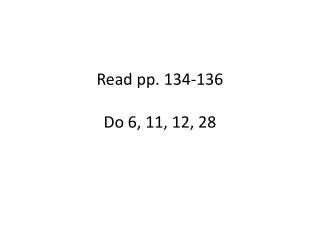DownloadDownload PresentationRead pp. 134-136 Do 6, 11, 12, 28

# Read pp. 134-136 Do 6, 11, 12, 28

Download Presentation## Read pp. 134-136 Do 6, 11, 12, 28

- - - - - - - - - - - - - - - - - - - - - - - - - - - E N D - - - - - - - - - - - - - - - - - - - - - - - - - - -
##### Presentation Transcript

1. Read pp. 134-136Do 6, 11, 12, 28

2. Pendula (or Pendulums, in the vernacular)

3. What is a pendulum? • Pendulum: Consists of a massive object called a bob suspended by a string. • What is it good for? • Keeping time • Galileo (what other options did he have?) • Clocks • Calculating acceleration due to gravity • Other, less obvious things • Verifying the rotation of the earth • Understanding Energy: Potential and Kinetic • Study of simple harmonic motion

4. Velocity, acceleration & the Pendulum

5. Conservation of Energy & The Pendulum Definitions Potential energy describes the possibility of converting stored energy into motion Kinetic energy describes the energy of motion Since (mass)energy can be neither created nor destroyed, potential and kinetic energy can be exchanged interchangeable This height indicates the distance the pendulum bob “falls” under the influence of gravity. It and gravity determine how much potential there is for motion We are back at the same height! And at ½ of a cycle. Kinetic energy is a measure of how fast the bob is moving.

6. Conservation of Energy & The Pendulum We are back at the same height! And at ½ of a cycle.

7. Kinetic and Potential Energy Kinetic Energy = ½ m v2 Potential energy = mg D h

8. With pendula, we are usually interested in the period What things might affect the period? - mass - angle - length - gravity - wind resistance What do you think the form of the equation might be?

9. Pendulum: Consists of a massive object called a bob suspended by a string • Pendula go through periodic motion as follows: Where: T = period, or the time to go through one cycle l = length of pendulum string g = acceleration of gravity • Note: • This formula is true for only small angles of θ. • What would you expect to find in this equation and is not there?

10. The motion of a pendulum is independent of the mass • As long as the mass is big enough so that air resistance can be ignored • As long as the mass of the string holding the pendulum is very small compared to the mass of the bob Where: T = period l = length of pendulum string g = acceleration of gravity

11. As we increase l, what happens? As we increase g, what happens?

12. As we increase the angle, what happens? As we increase the mass, what happens?

13. Examples: • What is the period of a pendulum on Earth with a length of 1m? • What is the period of a pendulum on Jupiter with a length of 1m? (ag = 3g) • What is the period of a pendulum on the moon with a length of 2m? (ag = 1/6g) • What is the length of a pendulum (on Earth) with a period of 10 sec? • What is the length of a pendulum (on Earth) with a length of 0.1 sec?

14. Examples(2): • What would the graph of period vs. length look like? • What would the graph of period2 versus length look like? • What would the graph of period2 versus g look like?